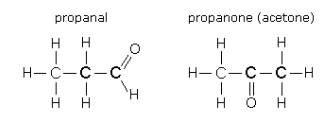# Question #b9f95

May 11, 2017

b) There can be isomeric pairs in (i) alcohols and ethers and in (ii) aldehydes and ketones.

#### Explanation:

a) Alkanes and cycloalkanes

These are not isomeric with each other.

Compare propane (${\text{C"_3"H}}_{8}$) and cyclopropane (${\text{C"_3"H}}_{6}$).They cannot be isomers because they have different molecular formulas.

The general formula for alkanes is ${\text{C"_text(n)"H}}_{\textrm{2 n + 2}}$, while the general formula for alkenes is ${\text{C"_text(n)"H}}_{\textrm{2 n}}$.

b) Oxygen-containing compounds

The classes are not isomeric with each other.

However, members from each class with the same number of carbon atoms can be isomers, depending on the position of the oxygen atom.

(i) Alcohols and ethers

Compare ethanol $\text{C"_2"H"_6"O}$ and dimethyl ether $\text{C"_2"H"_6"O}$.They are isomers because they have the same chemical formula but different arrangements of their atoms.

The general formula of alcohols and ethers is $\text{C"_text(n)"H"_text(2n+2)"O}$.

(ii) Aldehydes and Ketones

Compare propanal and $\text{C"_3"H"_6"O}$ and propanone $\text{C"_3"H"_6"O}$.They are isomers because they have the same chemical formula but different arrangements of their atoms.

The general formula of alcohols and ethers is $\text{C"_text(n)"H"_text(2n)"O}$.